# SAT Math Multiple Choice Question 992: Answer and Explanation

### Test Information

Question: 992

12.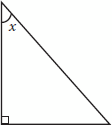In the triangle above, tan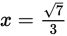. What is cos x?

• A.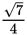• B.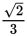• C.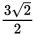• D.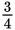Explanation:

D

Difficulty: Medium

Category: Additional Topics in Math / Trigonometry

Strategic Advice: Mark up the triangle with what you know. Then use SOH CAH TOA: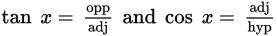.

Getting to the Answer: You're given that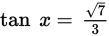, so label the side that is opposite angle x as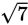, and label the side that is adjacent to angle x (the leg that touches it) 3: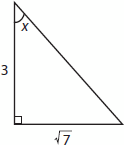Next, use the Pythagorean theorem to find the length of the hypotenuse: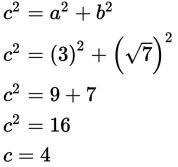Finally, use the definition of cosine: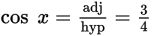, which is (D).